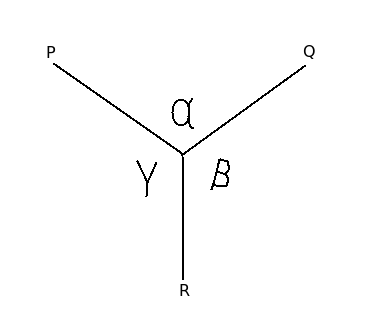# Lami's theorem

Q.  According to Lami's theorem, a body is in equilibrium condition if each force among three ____________ are proportional to sine angle between other two.
- Published on 18 Sep 15

a. coplanar, collinear forces
b. collinear, non-concurrent forces
c. coplanar, non-concurrent forces
d. coplanar, concurrent forces

#### Discussion

• Sravanthi   -Posted on 16 Dec 15
- According to Lami's theorem, a body is in equilibrium condition if each force among three coplanar, concurrent forces are proportional to sine angle between other two.The mathematical representation of Lami's theorem is given as follows:

(P / sin α) = (Q / sin β) = (R / sin γ)

This equation relates the magnitude of three coplanar, concurrent and non-collinear forces which maintain a body in static equilibrium.

## ➨ Post your comment / Share knowledgeEnter the code shown above:

(Note: If you cannot read the numbers in the above image, reload the page to generate a new one.)